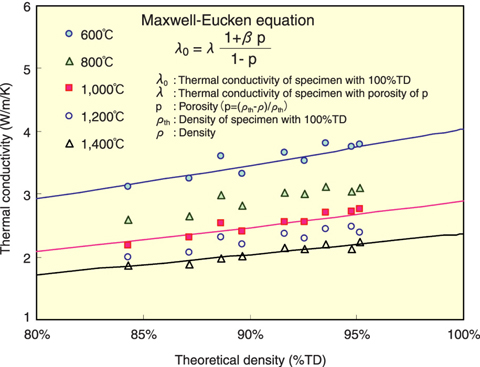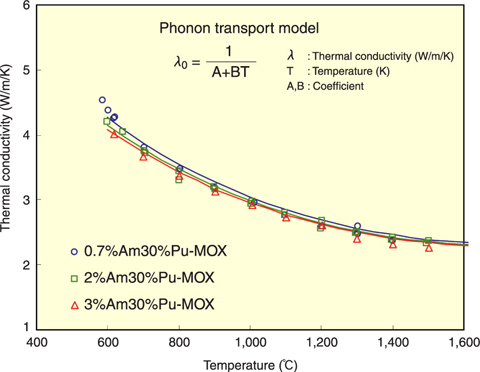# 12-5 Study on Physical Properties of Fast Reactor Fuel for Future Commenricialization

## - Study on Thermal Conductivities of Uranium-Plutonium Mixed Oxide Fuels -Fig.12-9 Dependency of thermal conductivity on density

The symbols are the thermal conductivities evaluated experimentally. Conductivity tends to decrease with decrease in density. Solid lines were calculated with the Maxwell-Eucken equation. The calculated values show a good agreement with experimental ones. This result shows that Maxwell-Eucken equation makes possible correction of density in the range from 85 to 95%TD.Fig.12-10 Dependency of thermal conductivity on Am-content

The symbols are the thermal conductivities evaluated experimentally. The thermal conductivities of MOX fuels containing Am decreased slightly with increase in Am-content. The thermal conductivities up to about 1500 K satisfied the classical phonon transport model, λ0 = (A＋BT)-1. The coefficient A increased linearly with an increase of the Am-content.

A sodium-cooled fast reactor using oxide fuel is considered the most practical reactor for the next-generation nuclear fuel cycle system. Research and development for practical use of this reactor is being carried out. In fuel design, evaluation of reactor and fuel characteristics is needed for operating this reactor at high linear heat rate, so that we can obtain large amounts of energy from the reactor, without melting the fuel. We can carry out rational fuel design by using data with high reliability. Thermal conductivity is one of the most important thermal physical properties in fuel design.

The thermal conductivity of uranium-plutonium mixed oxide (MOX) used as fast reactor fuel is changed by density, oxygen to metal ratio (O/M ratio), etc. The specifications of densities of this fuel are different according to the characteristics of reactors. The prototype fast-breeder reactor "MONJU" uses the low-density type fuel with theoretical density (%TD) of 85%. The density of this type of fuel is adjusted by controlling porosity. If the storage time between reprocessing of the irradiated fuel and MOX fuel loading into the reactor becomes long, a considerable amount of 241Am accumulates in the MOX fuel, which may affect its thermal and mechanical properties.

In this study, three kinds of MOX fuels with different Am-content were prepared, and their thermal conductivities were measured at temperatures from 600 to 1,500°C. From these results, the effects of Am-content (from 0.7 to 3%) and density (from 85 to 95 %TD) on thermal conductivity were clarified. Fig.12-9 and Fig.12-10 show the dependency of thermal conductivity on density and Am-content, respectively. From the analysis of these dependencies, the influences of density and Am-content on thermal conductivity could be described theoretically.

Some reports on thermal conductivity of MOX fuel have been published, but the ranges of Pu-content, density and O/M ratio of specimens were less than 20%, more than 90%TD and from 1.96 to 2.00 respectively. These measurement ranges are not sufficient for the thermal conductivity evaluation of fast reactor fuel in fuel design. In addition, there is no report that describes the influence of Am-content on thermal conductivity. In this study, the thermal conductivities of (U, Pu, Am)O2 solid solutions were measured as a function of density (85 to 95 TD%). The evaluation formula on the density dependency of thermal conductivity obtained in this measurement was confirmed to apply to low-density type fuel like that of MONJU . The thermal conductivities of (U, Pu, Am)O2 solid solutions were measured as functions of Am-content (0.7 to 3.1%) for the first time in this study. It was found that the thermal conductivities of MOX fuels decreased slightly with increasing Am-content in the range of a few percent. We are now investigating thermal conductivities of MOX fuels over wide ranges of temperature (to 2,000°C) and O/M ratio (from 1.91 to 2.00), and will proceed to carry out comprehensive tests for evaluation of fast reactor fuel characteristics in the future.

| | | | |